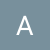LRU Cache Implementation

class LRUCache {

private Map<Integer, Node> map;
final Node head = new Node();
final Node tail = new Node();
private int capacity;
public LRUCache(int capacity) {
this.map = new HashMap<>(capacity);
this.capacity = capacity…

Implement HashMap in Java

to implement following functions in HashMap:

put(), get(), remove(), size(),keySet(),containsKey()

We will be using Arrays of LinkedList . Each linked List will represent a bucket. After hashing of keys, the key will be added to its corresponding bucket.

Our Node will look like this .

private class HMNode{…

Implementing Tries

class Trie {
class TrieNode{
Map<Character,TrieNode> children = new HashMap<>();
boolean isLeaf;
}
private TrieNode root;
/** Initialize your data structure here. */
public Trie() {
root= new TrieNode();
}
/** Inserts a word into the trie. */
public void insert(String word) {
TrieNode node = root;
for(char c : word.toCharArray()){
if(!node.children.containsKey(c)){

node.children.put(c,new TrieNode());
}
node = node.children.get(c);
}
node.isLeaf= true;
}
/** Returns if the word is in the trie. */
public boolean search(String word) {
TrieNode p = root;
for(char c : word.toCharArray()) {
if(!p.children.containsKey(c)) {
return false;
}
p = p.children.get(c);
}
return p.isLeaf;
}

/** Returns if there is any word in the trie that starts with the given prefix. */
public boolean startsWith(String prefix) {
TrieNode p = root;
for(char c : prefix.toCharArray()) {
if(!p.children.containsKey(c)) {
return false;
}
p = p.children.get(c);
}
return true;
}
}

Union Disjoint -Rank and Path Compression

class DSU{
//path compression and union by rank
int[] parent;
int[] rank;
int components;
public DSU(int components){
this.components=components;
parent = new int[components];
for(int i =0 ; i<components;i++){
parent[i]=i;//initially node is the parent of itself
}
rank = new int[components]; //initial rank=0

}

public int find(int node){
if(parent[node]!=node) {
parent[node]=find(parent[node]);
}
return parent[node];
}

public boolean union(int x, int y){
int px = find(x);
int py = find(y);
if(px==py) return false;
else{
else if (rank[px]<rank[py]) parent[px]=py;
else if(rank[px]>rank[py]) parent[py]=px;
else {
parent[py]=px;
rank[px]++;
}
componenets--;
}
return true;
}
}

From INORDER and POSTORDER

Given two integer arrays inorder and postorder where inorder is the inorder traversal of a binary tree and postorder is the postorder traversal of the same tree, construct and return the binary tree.

Algorithm:

In Postorder, the rightmost element is the root. Search this root in inorder array .Let that…

Inorder Predecessor and Successor in BST

There is BST given with root node with key part as integer only. You need to find the inorder successor and predecessor of a given key. In case, if the either of predecessor or successor is not found return null.

Algorithm:

For Predecesor:

1. One left and Extreme right.(If node has…

Insertion:

You are given the root node of a binary search tree (BST) and a value to insert into the tree. Return the root node of the BST after the insertion. It is guaranteed that the new value does not exist in the original BST.

Notice that there may exist multiple…

Target Sum

Given an array of non-negative integers and a target sum S.

Assign+ or — to every element such that their sum becomes S..

Input: nums is [1, 1, 1, 1, 1], S is 3.
Output: 5
Explanation:
-1+1+1+1+1 = 3
+1-1+1+1+1 = 3
+1+1-1+1+1 = 3
+1+1+1-1+1 = 3
+1+1+1+1-1 = 3…

Largest String after k deletions

Given a string of length n, return the largest string based on dictionary order, that can be obtained by erasing k characters from that string.

For two strings, x and y, each consisting of n characters, x is larger than y, based on dictionary order, when the first i-1 letters…

Given a non-empty array of length n with non-negative elements. Write a program to find and return the maximum sum of a contiguous

subarray of the given array such that no two elements are repeated in the subarray.

Input: 1 2 3 3 4 5 2 1
Output: 15
Explanation: Subarray from index 3 to 7 constitutes the sum 15(3+4+5+2+1)

Approach:

We will use two pointers i and j. We will use HashSet…